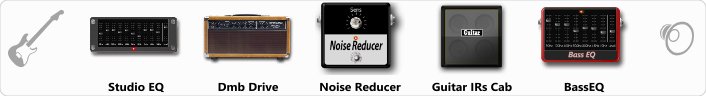# Dumble Clean

Discussion in 'ToneLib-GFX presets' started by Jean C.Guion, Sep 26, 2019.

1. Dumble Clean

Preset name: Dumble Clean

Effects chain:Effect: "Studio EQ" (Dynamics / Filter), active - "yes"
"31 Hz" = 11
"62 Hz" = -5
"125 Hz" = 1
"250 Hz" = -5
"500 Hz" = -4
"1 kHz" = 0
"2 kHz" = -12
"4 kHz" = -6
"8 kHz" = 11
"16 kHz" = 13
"above 16 kHz" = 0
"Level (dB)" = -9

Effect: "Dmb Drive" (Amp simulators), active - "yes"
"Gain" = 29
"Bass" = 100
"Middle" = 64
"Treble" = 34
"Presence" = 5
"Master" = 100
"Output" = 50
"Level (dB)" = 9

Effect: "Noise Reducer" (Dynamics / Filter), active - "yes"
"Sens" = 15
"Mode" = Hard

Effect: "Guitar IRs Cab" (Cabinets), active - "yes"
"Model" = Soldano Slant (4x12")
"Mic Position" = Middle
"Mic Distance" = Middle
"Low Cut (Hz)" = 39
"Hi Cut (kHz)" = 4.0
"Mix" = 100
"Level (dB)" = 11

Effect: "BassEQ" (Dynamics / Filter), active - "yes"
"50 Hz" = 15
"120 Hz" = 6
"400 Hz" = -4
"500 Hz" = 4
"800 Hz" = -8
"4.5 kHz" = 6
"10 kHz" = 4
"Level (dB)" = 0

Note: You will need to download and install the ToneLib-GFX software to use the preset.

File size:
530 bytes
Views:
4,140